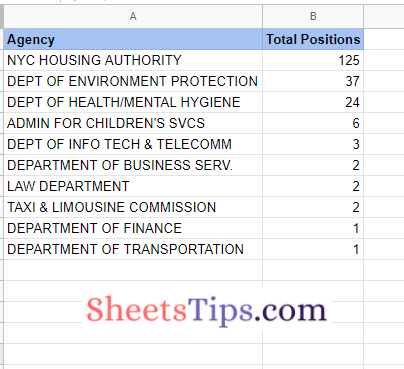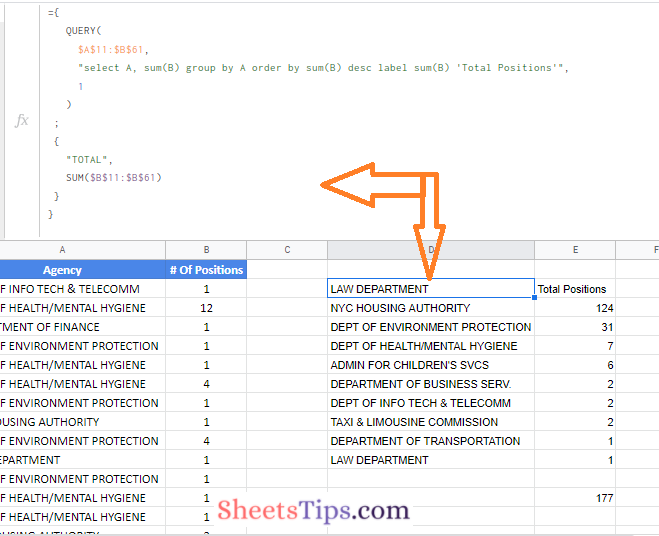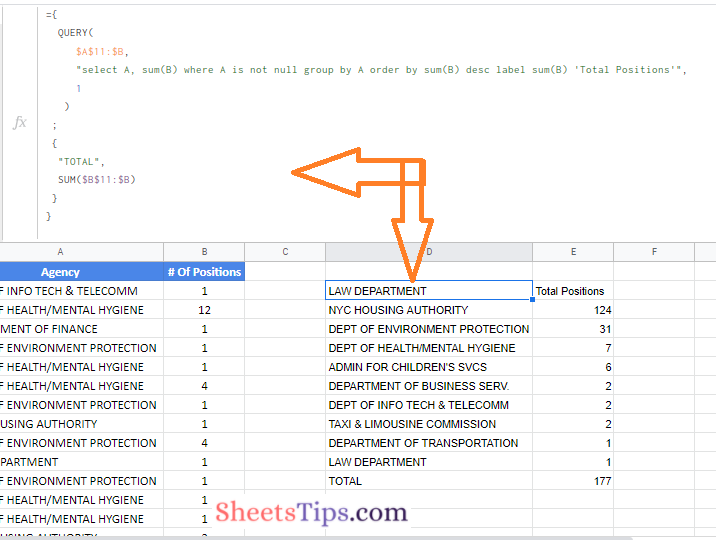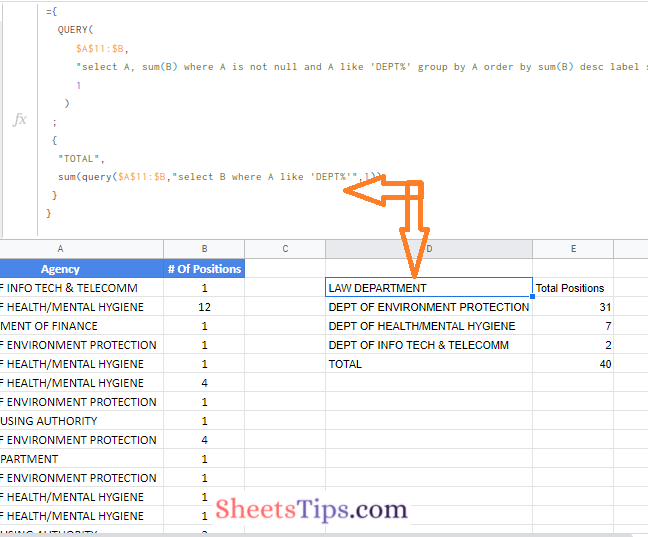# How To Add a Total Row in Query Function Table in Google Sheets?

This tutorial will talk everything about how to add total rows to the tables generated using the query function. Instead of using the array formulas, on this page, we will use the pair of curly braces with the help of which we will add the total row in a query function.

Well, most of you will be thinking that we can simply write the word Total and use the SUM(range) formula to draw the total results. One must note that this is the manual step. In order to create the dynamic total row in Google Sheets, we can simply use the {….} notation in an array formula. Let us understand how to do this in Spreadsheet using the Google Sheets Tips and Tricks. Read further to find more.

We will use the same example which we have used in the Onion method for complex formulas.

In that example, what we would want to do is merge all of the organization rows into a single row with a total count for that organization. We are “grouping” our data into the categories in column A, then adding up all of the values in column B that belong to each group.

To do so, we combine the number of positions data for each agency using the QUERY function and a group by clause. The formula which we used for achieving this is as follows:

=QUERY(\$A\$11:\$B\$61,”select A, sum(B) group by A order by sum(B) desc label sum(B) ‘Total Positions'”,1)

By applying this formula in Google Sheets we would have got the output as shown in the image below.Now let us discuss how to add dynamic total in the Google Sheets Query function.

### How Do You Add a Total Row in a Query Function?

So, we are doing the same thing as the simple example before, which is to create two different tables. One is a summary table, such as the one seen above, and the other is a total row, which we merge using an array formula.

The steps to get this done in Google Sheets are as follows:

• 1st Step: Move to the cell where you want to add a total row.
• 2nd Step: Now modify the previously used formula with the use of the Total function. So our formula here is
 ={ QUERY( \$A\$11:\$B\$61, “select A, sum(B) group by A order by sum(B) desc label sum(B) ‘Total Positions'”, 1 ) ; { “TOTAL”, SUM(\$B\$11:\$B\$61) } }
• 3rd Step: Press the “Return” key and now you will see the results as shown below.### Last Step to Make the Total Row in Query Function Dynamic

This is the final step where we want to generate the query function into a dynamic. As the table expands or contracts as we add or delete data, the total row goes up or down without any manual changes.

There are two things we must achieve:

1. Change the range to include all of columns A and B, and
2. Change the QUERY function to eliminate the blank row that appears after step 1.

Remove the references from the range so that all columns A and B are included.Then add a WHERE clause to the QUERY function to get away from all the blank rows in column A. So our formula here is

 ={ QUERY( \$A\$11:\$B, “select A, sum(B) where A is not null and A like ‘DEPT%’ group by A order by sum(B) desc label sum(B) ‘Total Positions'”, 1 ) ; { “TOTAL”, SUM(QUERY(\$A\$11:\$B,”select B where A like ‘DEPT%'”,1)) } }

Enter the above formula and press the return key and you will see the results.